# US10268026B2 - Optical system, optical apparatus and method for manufacturing the optical system - Google Patents

Optical system, optical apparatus and method for manufacturing the optical system Download PDF

## Info

Publication number
US10268026B2
US10268026B2 US14/381,277 US201314381277A US10268026B2 US 10268026 B2 US10268026 B2 US 10268026B2 US 201314381277 A US201314381277 A US 201314381277A US 10268026 B2 US10268026 B2 US 10268026B2
Authority
US
United States
Prior art keywords
lens
object
cemented
denotes
optical system
Prior art date
Legal status (The legal status is an assumption and is not a legal conclusion. Google has not performed a legal analysis and makes no representation as to the accuracy of the status listed.)
Active
Application number
US14/381,277
Other versions
US20150085377A1 (en
Inventor
Atsushi Suzuki
Current Assignee (The listed assignees may be inaccurate. Google has not performed a legal analysis and makes no representation or warranty as to the accuracy of the list.)
Nikon Corp
Original Assignee
Nikon Corp
Priority date (The priority date is an assumption and is not a legal conclusion. Google has not performed a legal analysis and makes no representation as to the accuracy of the date listed.)
Filing date
Publication date
Priority to JP2012042330A priority Critical patent/JP5903932B2/en
Priority to JP2012-042330 priority
Priority to JP2012-050009 priority
Priority to JP2012050009A priority patent/JP5903937B2/en
Application filed by Nikon Corp filed Critical Nikon Corp
Priority to PCT/JP2013/001081 priority patent/WO2013128882A1/en
Assigned to NIKON CORPORATION reassignment NIKON CORPORATION ASSIGNMENT OF ASSIGNORS INTEREST (SEE DOCUMENT FOR DETAILS). Assignors: SUZUKI, ATSUSHI
Publication of US20150085377A1 publication Critical patent/US20150085377A1/en
Application granted granted Critical
Publication of US10268026B2 publication Critical patent/US10268026B2/en
Application status is Active legal-status Critical
Anticipated expiration legal-status Critical

• 230000003287 optical Effects 0 abstract claims description title 214
• 230000014509 gene expression Effects 0 abstract claims description 87
• 230000004075 alteration Effects 0 description 176
• 206010010071 Coma Diseases 0 description 41
• 201000009310 astigmatism Diseases 0 description 16
• 239000003570 air Substances 0 description 14
• 238000006243 chemical reaction Methods 0 description 10
• 230000000694 effects Effects 0 description 10
• -1 distortion Diseases 0 description 8
• 238000009740 moulding (composite fabrication) Methods 0 description 8
• 230000000875 corresponding Effects 0 description 7
• 230000003247 decreasing Effects 0 description 7
• 230000001965 increased Effects 0 description 6
• 239000000463 materials Substances 0 description 4
• 239000007787 solids Substances 0 description 4
• 238000003384 imaging method Methods 0 description 3
• 102100002841 ST20 Human genes 0 description 2
• 230000001276 controlling effects Effects 0 description 2
• 239000004973 liquid crystal related substances Substances 0 description 2
• 230000002829 reduced Effects 0 description 2
• 239000010408 films Substances 0 description 1
• 230000015654 memory Effects 0 description 1
• 239000000203 mixtures Substances 0 description 1
• 230000011514 reflex Effects 0 description 1

## Images

•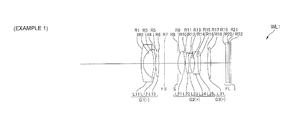•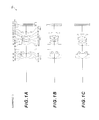•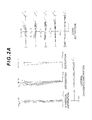•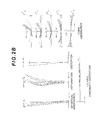•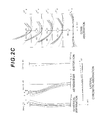•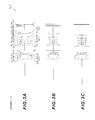•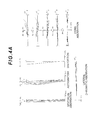•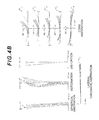•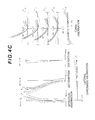•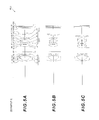•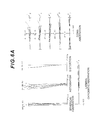••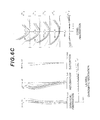•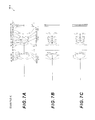•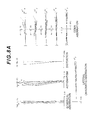•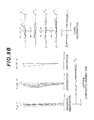•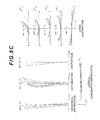••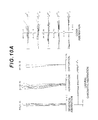•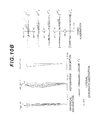•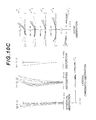••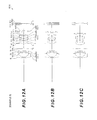•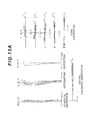•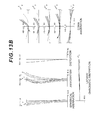•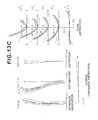•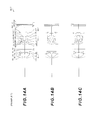•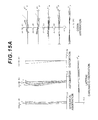•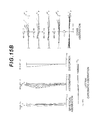•••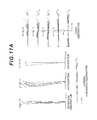•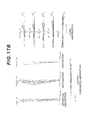•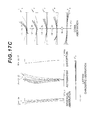•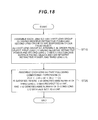•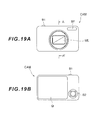•## Classifications

• GPHYSICS
• G02OPTICS
• G02BOPTICAL ELEMENTS, SYSTEMS, OR APPARATUS
• G02B13/00Optical objectives specially designed for the purposes specified below
• G02B13/04Reversed telephoto objectives
• GPHYSICS
• G02OPTICS
• G02BOPTICAL ELEMENTS, SYSTEMS, OR APPARATUS
• G02B13/00Optical objectives specially designed for the purposes specified below
• G02B13/001Miniaturised objectives for electronic devices, e.g. portable telephones, webcams, PDAs, small digital cameras
• G02B13/0015Miniaturised objectives for electronic devices, e.g. portable telephones, webcams, PDAs, small digital cameras characterised by the lens design
• G02B13/002Miniaturised objectives for electronic devices, e.g. portable telephones, webcams, PDAs, small digital cameras characterised by the lens design having at least one aspherical surface
• G02B13/0045Miniaturised objectives for electronic devices, e.g. portable telephones, webcams, PDAs, small digital cameras characterised by the lens design having at least one aspherical surface having five or more lenses
• GPHYSICS
• G02OPTICS
• G02BOPTICAL ELEMENTS, SYSTEMS, OR APPARATUS
• G02B13/00Optical objectives specially designed for the purposes specified below
• G02B13/18Optical objectives specially designed for the purposes specified below with lenses having one or more non-spherical faces, e.g. for reducing geometrical aberration
• YGENERAL TAGGING OF NEW TECHNOLOGICAL DEVELOPMENTS; GENERAL TAGGING OF CROSS-SECTIONAL TECHNOLOGIES SPANNING OVER SEVERAL SECTIONS OF THE IPC; TECHNICAL SUBJECTS COVERED BY FORMER USPC CROSS-REFERENCE ART COLLECTIONS [XRACs] AND DIGESTS
• Y10TECHNICAL SUBJECTS COVERED BY FORMER USPC
• Y10TTECHNICAL SUBJECTS COVERED BY FORMER US CLASSIFICATION
• Y10T29/00Metal working
• Y10T29/49Method of mechanical manufacture
• Y10T29/49826Assembling or joining

## Abstract

Provided is an optical system having, in order from an Object, a first lens group (G1) having negative refractive power and a second lens group (G2), wherein the first lens group (G1) includes, in order from the object, a first negative lens (L11) and a second negative lens (L12) having a concave surface facing the object, and the following conditional expression (1) is satisfied:
7.94≤(−fn12)/f<48.00  (1)
• where fn12 denotes a focal length of the second negative lens (L12), and f denotes a focal length of the entire optical system.

## Description

TECHNICAL FIELD

The present invention relates to an optical system having a wide angle and large aperture that is suitable for an imaging optical system of a digital camera, film camera, video camera or the like.

TECHNICAL BACKGROUND

A main stream of zoom lenses of compact digital cameras currently available includes a type where the lens barrel collapses into the camera when the camera is not used. This type where the lens barrel collapses into the camera when the camera is not used has been proposed not only for a zoom lens, but also for a wide angle lens with a fixed focal point, of which focal length, with respect to an infinite object point, does not change (e.g. see Patent Document 1).

PRIOR ARTS LIST Patent Document

Patent Document 1: Japanese Laid-Open Patent Publication No. 2008-40033(A)

SUMMARY OF THE INVENTION Problems to be Solved by the Invention

A conventional wide angle lens with a fixed focal point is compact and has a wide angle of view, but has about a 4.0 Fno, which is dark.

With the foregoing in view, it is an object of the present invention to provide an optical system and an optical apparatus of which the lens barrel can collapse into a camera when the camera is not used, and which still has a small size, a wide angle of view and a large aperture, and a method for manufacturing the optical system.

Means to Solve the Problems

To achieve this object, an optical system according to this invention has, in order from an object, a first lens group having negative refractive power; and a second lens group, wherein the first lens group includes, in order from the object, a first negative lens and a second negative lens having a concave surface facing the object, and the following conditional expression is satisfied.
7.94≤(−fn12)/f<48.00

where fn12 denotes a focal length of the second negative lens, and f denotes a focal length of the entire optical system.

In the optical system according to this invention, it is preferable that the first lens group includes, in order from the object, the first negative lens, the second negative lens and a positive lens.

In the optical system according to this invention, it is preferable that the following conditional expression is satisfied.
−30.00<νp13−νn12<30.00

where νp13 denotes an Abbe number of the positive lens with respect to the d-line, and νn12 denotes an Abbe number of the second negative lens with respect to the d-line.

In the optical system according to this invention, it is preferable that the second negative lens is a meniscus lens.

In the optical system according to this invention, it is preferable that the first negative lens, the second negative lens and the positive lens are all single lenses.

In the optical system according to this invention, it is preferable that the second lens group has positive refractive power.

In the optical system according to this invention, it is preferable that an aperture stop is disposed closer to the object than the second lens group.

In the optical system according to this invention, it is preferable that at least one surface of the first negative lens is aspherical.

An optical apparatus according to this invention includes one of the above optical systems.

A method for manufacturing an optical system according to this invention is a method for manufacturing an optical system having, in order from an object, a first lens group having negative refractive power and a second lens group, wherein the first lens group includes, in order from the object, a first negative lens and a second negative lens having a concave surface facing the object, and each lens is assembled in a lens barrel such that the following conditional expression is satisfied.
7.94≤(−fn12)/f<48.00

where fn12 denotes a focal length of the second negative lens, and f denotes a focal length of the entire optical system.

An optical system according to this invention has, in order from an object: a first lens group having negative refractive power; and a second lens group, wherein the first lens group includes, in order from the object, a first lens having negative refractive power, a second lens which has a concave surface facing the object and has negative refractive power, and a third lens, and the following conditional expression is satisfied.
−23.0<νd3−νd2<24.2

where νd3 denotes an Abbe number of the third lens with respect to the d-line, and νd2 denotes an Abbe number of the second lens with respect to the d-line.

In the optical system according to this invention, it is preferable that the following conditional expression is satisfied.
fL1/FL2<0.2

where fL1 denotes a focal length of the first lens, and fL2 denotes a focal length of the second lens.

In the optical system according to this invention, it is preferable that the following conditional expression is satisfied.
2.55<(−R21)/R12

where R21 denotes a radius of curvature of the object side surface of the second lens, and R12 denotes a radius of curvature of an image side surface of the first lens, and the radius of curvatures R21 and R12 are positive when the convex surface faces the object.

In the optical system according to this invention, it is preferable that the third lens has positive refractive power.

In the optical system according to this invention, it is preferable that the first lens, the second lens and the third lens are all single lenses.

In the optical system according to this invention, it is preferable that the second lens group has positive refractive power.

In the optical system, according to this invention, it is preferable that an aperture stop is disposed closer to the object than the second lens group.

In the optical system according to this invention, it is preferable that at least one surface of the first lens is aspherical.

An optical apparatus according to this invention includes one of the above optical systems.

A method for manufacturing an optical system according to this invention is a method for manufacturing an optical system having, in order from an object, a first lens group having negative refractive power and a second lens group, wherein the first lens group includes, in order from the object, a first lens having negative refractive power, a second lens which has a concave surface facing the object and has negative refractive power, and a third lens, and each lens is assembled in a lens barrel such that the following conditional expression is satisfied.
−23.0<νd3−νd2<24.2

where νd3 denotes an Abbe number of the third lens with respect to the d-line, and νd2 denotes an Abbe number of the second lens with respect to the d-line.

This invention can provide an optical system and an optical apparatus of which lens barrel can collapse into the camera when the camera is not used, and which still has a small size, a wide angle of view and a large aperture, and a method for manufacturing the optical system.

BRIEF DESCRIPTION OF THE DRAWINGS

FIG. 1 are cross-sectional views depicting a configuration of an optical system according to Example 1, where FIG. 1A shows an arrangement upon focusing on infinity (β=0.0000), FIG. 1B shows an arrangement upon focusing on a point at an intermediate distance (β=−0.0359), and FIG. 1C shows an arrangement upon focusing on a nearest point (β=−0.1790);

FIG. 2 are graphs showing various aberrations of the optical system according to Example 1, where FIG. 2A shows an aberration upon focusing on infinity (β=0.0000), FIG. 2B shows an aberration upon focusing on a point at an intermediate distance (β=−0.0359), and FIG. 2C shows an aberration upon focusing on a nearest point (β=−0.1790);

FIG. 3 are cross-sectional views depicting a configuration of an optical system according to Example 2, where FIG. 3A shows an arrangement upon focusing on infinity (β=0.0000), FIG. 3B shows an arrangement upon focusing on a point at an intermediate distance (β=−0.0359), and FIG. 3C shows an arrangement upon focusing on a nearest point (β=−0.1808);

FIG. 4 are graphs showing various aberrations of the optical system according to Example 2, where FIG. 4A shows an aberration upon focusing on infinity (β=0.0000), FIG. 4B shows an aberration upon focusing on a point at an intermediate distance (β=−0.0359), and FIG. 4C shows an aberration upon focusing on a nearest point (β=−0.1808);

FIG. 5 are cross-sectional views depicting a configuration of an optical system according to Example 3, where FIG. 5A shows an arrangement upon focusing on infinity (β=0.0000), FIG. 5B shows an arrangement upon focusing on a point at an intermediate distance (β=−0.0358), and FIG. 5C shows an arrangement upon focusing on a nearest point (β=−0.1772);

FIG. 6 are graphs showing various aberrations of the optical system according to Example 3, where FIG. 6A shows an Aberration upon focusing on infinity (β=0.0000), FIG. 6B shows an Aberration upon focusing on a point at an intermediate distance (β=−0.0358), and FIG. 6C shows an aberration upon focusing on a nearest point (β=−0.1772);

FIG. 7 are cross-sectional views depicting a configuration of an optical system according to Example 4, where FIG. 7A shows an arrangement upon focusing on infinity (β=0.000), FIG. 7B shows an arrangement upon focusing on a point at an intermediate distance (β=−0.009), and FIG. 7C shows an arrangement upon focusing on a nearest point (β=−0.036);

FIG. 8 are graphs showing various aberrations of the optical system according to Example 4, where FIG. 8A shows an Aberration upon focusing on infinity (β=0.000), FIG. 8B shows an aberration upon focusing on a point at an intermediate distance (β=−0.009), and FIG. 8C shows an aberration upon focusing on a nearest point (β=−0.036);

FIG. 9 are cross-sectional views depicting a configuration of an optical system according to Example 5, where FIG. 9A shows an arrangement upon focusing on infinity (β=0.000), FIG. 9B shows an arrangement upon focusing on a point at an intermediate distance (β=−0.009), and FIG. 9C shows an arrangement upon focusing on a nearest point (β=−0.036);

FIG. 10 are graphs showing various aberrations of the optical system according to Example 5, where FIG. 10A shows an aberration upon focusing on infinity (β=0.000), FIG. 10B shows an aberration upon focusing on a point at an intermediate distance (β=−0.009), and FIG. 10C shows an aberration upon focusing on a nearest point (β=−0.036);

FIG. 11 is a flow chart depicting a method for manufacturing the optical system according to Embodiment 1;

FIG. 12 are cross-sectional views depicting a configuration of an optical system according to Example 6, where FIG. 12A shows an arrangement upon focusing on infinity (β=0.000), FIG. 12B shows an arrangement upon focusing on a point at an intermediate distance (β=−0.036), and FIG. 12C shows an arrangement upon focusing on a nearest point (β=−0.179);

FIG. 13 are graphs showing various aberrations of the optical system according to Example 6, where FIG. 13A shows an aberration upon focusing on infinity (β=0.000), FIG. 13B shows an aberration upon focusing on a point at an intermediate distance (β=−0.036), and FIG. 13C shows an aberration upon focusing on a nearest point (β=−0.179);

FIG. 14 are cross-sectional views depicting a configuration of an optical system according to Example 7, where FIG. 14A shows an arrangement upon focusing on infinity (β=0.0000), FIG. 14B shows an arrangement upon focusing on a point at an intermediate distance (β=−0.009), and FIG. 14C shows an arrangement upon focusing on a nearest point (β=−0.036);

FIG. 15 are graphs showing various aberrations of the optical system according to Example 7, where FIG. 15A shows an aberration upon focusing on infinity (β=0.000), FIG. 15B shows an Aberration upon focusing on a point at an intermediate distance (β=−0.009), and FIG. 15C shows an aberration upon focusing on a nearest point (β=−0.036);

FIG. 16 are cross-sectional views depicting a configuration of an optical system according to Example 8, where FIG. 16A shows an arrangement upon focusing on infinity (β=0.000), FIG. 16B shows an arrangement upon focusing on a point at an intermediate distance (β=−0.009), and FIG. 16C shows an arrangement upon focusing on a nearest point (β=−0.036);

FIG. 17 are graphs showing various aberrations of the optical system according to Example 8, where FIG. 17A shows an aberration upon focusing on infinity (β=0.000), FIG. 17B shows an aberration upon focusing on a point at an intermediate distance (β=−0.009), and FIG. 17C shows an aberration upon focusing on a nearest point (β=−0.036);

FIG. 18 is a flow chart depicting a method for manufacturing the optical system according to Embodiment 2;

FIG. 19 shows a digital camera (optical apparatus) including the optical system according to Example 1, where FIG. 19A is a front view and FIG. 19B is a rear view; and

FIG. 20 is a cross-sectional view along the A-A′ line in FIG. 19A.

DESCRIPTION OF THE EMBODIMENTS

Embodiments of the present invention will now be described with reference to the drawings. Embodiment 1 of the present invention will be described first with reference to FIG. 1 to FIG. 11. Then Embodiment 2 of the present invention will be described with reference to FIG. 12 to FIG. 18. Finally optical apparatuses according to these embodiments will be described with reference to FIG. 19 and FIG. 20

(Embodiment 1)

As illustrated in FIG. 1, an optical system according to Embodiment 1 has, in order from an object, a first lens group G1 having negative refractive power, and a second lens group G2, wherein the first lens group G1 includes, in order from the object, a first negative lens L11, and a second negative lens L12 having a concave surface facing the object, and the following conditional expression (1) is satisfied.
7.94≤(−fn12)/f<48.00  (1)

where fn12 denotes a focal length of the second negative lens L12, and f denotes a focal length of the entire optical system.

Generally in the design of an imaging optical system, such as a photograph lens, it is difficult to make the angle of view wider and the aperture larger without changing the size of the optical system very much. As the aperture becomes larger, it becomes more difficult to correct spherical aberration, to correct both the meridional coma aberration and the sagittal coma aberration, and to correct both coma aberration and astigmatism. If the angle of view is made wider without increasing the size of the optical system, it becomes difficult to correct spherical aberration, astigmatism and various chromatic aberrations. However in the case of the optical system having the above configuration according to this embodiment, the lens barrel can collapse in the camera when the camera is not used, but the camera can be compact. Further, while implementing a large aperture of about Fno 2.0 and a wide angle of view of about 75°, the sagittal coma aberration can be decreased without worsening the meridional coma aberration.

The conditional expression (1) specifies the focal length of the second negative lens L12 constituting the first lens group G1. By satisfying the conditional expression (1), sagittal coma aberration can be decreased without worsening the meridional coma Aberration. If the upper limit value of conditional expression (1) is exceeded, the absolute value of the refractive power of the second negative lens L12 becomes too large. As a result, the absolute value of the radius of curvature of the object side surface of the second negative lens L12 increases, whereby sagittal coma aberration worsens, and correcting this becomes difficult. If the lower limit value of conditional expression (1) is not reached, the absolute value of the refractive power of the second negative lens L12 becomes too small. And as a result, the absolute value of the radius of curvature of the object side surface of the second negative lens L12 becomes small, which is effective to correct the sagittal coma aberration, however the meridional coma aberration worsens, and correcting this becomes difficult.

To demonstrate the effect of this embodiment with certainty, it is preferable that the upper limit value of conditional expression (1) is 40.00. To demonstrate the effect of this embodiment with greater certainty, it is preferable that the upper limit value of the conditional expression (1) is 30.00. To demonstrate the effect of this embodiment with even greater certainty, it is preferable that the upper limit value of conditional expression (1) is 20.00. To demonstrate the effect of this embodiment with even greater certainty, it is preferable that the upper limit value of the conditional expression (1) is 15.00. To demonstrate the effect of this embodiment with certainty, it is preferable that the lower limit value of conditional expression (1) is 0.85.

In the optical system according to Embodiment 1, it is preferable that the first lens group G1 includes, in order from the object, a first negative lens L11, a second negative lens L12 and a positive lens L13. By this configuration, distortion and lateral chromatic aberration can be corrected satisfactorily.

In the optical system according to Embodiment 1, it is preferable that the following conditional expression (2) is satisfied.
−30.00<νp13−νn12<30.00  (2)

where νp13 denotes an Abbe number of the positive lens L13 with respect to the d-line, and νn12 denotes an Abbe number of the second negative lens L12 with respect to the d-line.

The conditional expression (2) specifies the difference between the Abbe number of the second negative lens L12 and the Abbe number of the positive lens L13 constituting the first lens group G1. By satisfying the conditional expression (2), both the longitudinal chromatic aberration and the lateral chromatic aberration can be corrected satisfactorily. If the upper limit value of the conditional expression (2) is exceeded, the Abbe number of the second negative lens L12 becomes too small with respect to the Abbe number of the positive lens L13, therefore correction of the longitudinal chromatic aberration becomes difficult. If the lower limit value of the conditional expression (2) is not reached, the Abbe number of the second negative lens L12 becomes too large with respect to the Abbe number of the positive lens L13, therefore correction of the lateral chromatic aberration becomes difficult.

To demonstrate the effect of the embodiment with certainty, it is preferable that the upper limit value of the conditional expression (2) is 27.00. To demonstrate the effect of this embodiment with greater certainty, it is preferable that the upper limit value of the conditional expression (2) is 25.00. To demonstrate the effect of this embodiment with certainty, it is preferable that the lower limit value of the conditional expression (2) is −27.00. To demonstrate the effect of this embodiment with greater certainty, it is preferable that the lower limit value of the conditional expression (2) is −24.00.

In the optical system according to Embodiment 1, it is preferable that the second negative lens L12 is a meniscus lens having a concave surface facing the object. By this configuration, sagittal coma aberration can be corrected satisfactorily.

In the optical system according to Embodiment 1, it is preferable that the first negative lens L11, the second negative lens L12 and the positive lens L13 constituting the first lens group G1 are all single lenses. By this configuration, distortion and curvature of field can be corrected satisfactorily.

In the optical system according to Embodiment 1, it is preferable that the second lens group G2 has positive refractive power. By using a so called “retro focus type”, where the second lens group G2 having positive refractive power is disposed closer to the image than the first lens group G1 having negative refractive power, compactness with a wide angle of view can be implemented while controlling aberrations (especially curvature of field).

In the optical system according to Embodiment 1, it is preferable that an aperture stop S is disposed closer to the object than the second lens group G2. By this configuration, distortion and curvature of field can be corrected satisfactorily, while decreasing the effective diameter of the lens closest to the object, that is, the first negative lens L11 (of the first lens group G1). Furthermore, it is possible to decrease the thickness of the lens barrel in a state where the lens barrel collapses into the camera when the camera is not used, and a slimmer camera can be implemented.

In the optical system according to Embodiment 1, it is preferable that at least one surface of the first negative lens L11 is aspherical. If an aspherical surface is used for the first negative lens L11, where abaxial light passes through a position distant from the optical axis, then curvature of field and astigmatism can be corrected satisfactorily, and aberrations of the entire optical system can be corrected satisfactorily. Normally if achieving a wider angle of view is attempted in an optical system of which the first lens group G1 closest to the object has negative refractive power, negative refractive power of the first lens group G1 must be increased, which makes the correction of aberrations difficult. However if at least one surface of the first negative lens L11 constituting the first lens group G1 is aspherical, as in the case of the optical system of this embodiment, this problem can be solved. Aberrations can be corrected by increasing a number of lenses constituting the first lens group G1, but if a number of lenses of the first lens group G1 increases, the thickness of the lens barrel increases when the camera is not used, that is, when the lens barrel is collapsed, which makes downsizing impossible.

According to the above mentioned optical system of Embodiment 1, an optical system where the lens barrel can collapse into the camera when the camera is not used, but where the camera is still compact, and has a wide angle of view (angle of view: about) 75° and a large aperture (Fno: about 2.0), can be implemented.

Now a method for manufacturing the optical system will be described with reference to FIG. 11. First the first lens group G1 and the second lens group G2 are assembled in the lens barrel in order from the object (step ST10). In this assembly step, each lens is arranged so that the first lens group G1 has negative refractive power. Then each lens is assembled in the lens barrel so that the first lens group G1 includes, in order from the object, the first negative lens L11 and the second negative lens L12 having a concave surface facing the object, and the following conditional expression (1) is satisfied, where fn12 denotes a focal length of the second negative lens L12, and f denotes a focal length of the entire optical system (step ST20).
7.94≤(−fn12)/f<48.00  (1)

An example of the lens configuration according to Embodiment 1 is, as illustrated in FIG. 1, the first lens group G1 that includes, in order from the object, a negative meniscus lens L11 having a convex surface facing the object (corresponding to the first negative lens), a negative meniscus lens L12 having a concave surface facing the object (corresponding to the second negative lens), and a biconvex positive lens L13, so that the entire lens group has negative refractive power. The second lens group G2 includes, in order from the object, a biconvex positive lens L21, a cemented lens of a biconvex positive lens L22 and a biconcave negative lens L23, and a cemented lens of a biconcave negative lens L24 and a biconvex positive lens L25, so that the entire lens group has positive refractive power. The third lens group G3 includes a biconvex positive lens L31, so that the entire lens group has positive refractive power.

According to the above mentioned method for manufacturing the optical system according to Embodiment 1, an optical system where the lens barrel can collapse into the camera when the camera is not used, but where the camera is still compact, and has a wide angle of view (angle of view: about 75°) and a large aperture (Fno: about 2.0), can be implemented.

Each example of Embodiment 1 will now be described with reference to the drawings. Table 1 to Table 5 shown below list the data of Example 1 to Example 5.

In each example, the aberration characteristics are calculated with respect to the C-line (wavelength: 656.2730 nm), the d-line (wavelength: 587.5620 nm), the F-line (wavelength: 486.1330 nm), and the g-line (wavelength: 435.8350 nm).

In [Lens Data] in each table, the surface number is the sequential number of the optical surface counted from the object side in the light traveling direction, R denotes the radius of curvature of each optical surface, D denotes a distance from each optical surface to the next optical surface (or image plane) on the optical axis, nd denotes a refractive index of the material of the optical member with respect to the d-line, and νd denotes an Abbe number of the material of the optical member with respect to the d-line. The object surface indicates the surface of the object, (variable) indicates a variable surface distance, “∞” in the radius of curvature indicates a plane or an aperture, (stop FS) indicates a flare-cut stop FS, (stop S) indicates an aperture stop S, and image plane indicates an image plane I. The refractive index of air “1.000000” is omitted. If the optical surface is aspherical, * is affixed to the surface number, and the paraxial radius of curvature is shown in the column of the radius of curvature R.

In [Aspherical Data] in each table, a form of the aspherical surface shown in [Lens Data] is indicated by the following expression (a). X(y) denotes a distance in the optical axis direction from a tangential plane at a vertex of the aspherical surface to a position on the aspherical surface at height y, r denotes a radius of curvature (paraxial radius of curvature) of the reference spherical surface, K denotes a conical coefficient, and Ai denotes an aspherical coefficient of the i-th order. “E-n” indicates “×10−n”. For example, 1.234E-05=1.234×10−5.
X(y)=(y 2 /r)/{1+(1−κ×y 2 /r 2)1/2 }+Ay 4 +Ay 6 +Ay 8 +A10×y 10  (a)

In [Various Data], β denotes a photographic magnification at each focal position, f denotes a focal length of the entire optical system, FNO denotes an F number, ω denotes a half angle of view (maximum incident angle, unit: °), Y denotes an image height, TL denotes a total length of the optical system, Bf denotes a distance from an image side surface of an optical member disposed closest to the image to a paraxial image plane, and Bf (air conversion) denotes a distance from the last optical surface to the paraxial image plane converted into air.

In [Variable Surface Distance Data], β denotes a photographic magnification at each focal position, and Di denotes a variable surface distance of the i-th surface.

In [Conditional Expression], a value corresponding to each conditional expression (1) and (2) is shown.

In all the data values herein below, “mm” is normally used as the unit of focal length f, radius of curvature R, surface distance D and other lengths if not otherwise specified, but the unit is not limited to “mm”, and another appropriate unit may be used, since an equivalent optical performance is obtained even if the optical system is proportionally expanded or proportionally reduced.

This description on the table is the same for all examples, and is therefore omitted herein below.

EXAMPLE 1

Example 1 will be described with reference to FIG. 1, FIG. 2 and Table 1. As illustrated in FIG. 1, the optical system WL1 according to Example 1 has, in order from an object, a first lens group G1 having negative refractive power, a flare-cut stop FS, an aperture stop S, a second lens group G2 having positive refractive power, a third lens group G3 having positive refractive power, and a filter group FL.

The first lens group G1 includes, in order from the objet, a negative meniscus lens L11 having a convex surface facing the object, a negative meniscus lens L12 having a concave surface facing the object, and a biconvex positive lens L13. The image side lens surface of the negative lens L11 is aspherical.

The second lens group G2 includes, in order from the object, a biconvex positive lens L21, a cemented lens of a biconvex positive lens L22 and a biconcave negative lens L23 and a cemented lens of a biconcave negative lens L24 and a biconvex positive lens L25. The image side lens surface of the positive lens L25 is aspherical.

The third lens group G3 is constituted by a biconvex positive lens L31.

The filter group FL is constituted by a low-pass filter, an infrared cut-off filter or the like, in order to cut off the spatial frequency exceeding the critical resolution of a solid-state picture element (e.g. CCD, CMOS) disposed on the image plane I.

In the optical system WL1 of Example 1 having this configuration, it is preferable that focusing from an object at infinity to an object at a finite distance with about magnification β=−0.036 is performed by moving the third lens group G3 along the optical axis. It is preferable that focusing on an object at a closer distance with magnification β greater than −0.036 is performed by moving the second lens group G2 and the third lens group G3 respectively along the optical axis.

Table 1 shows each data value of Example 1. The surface numbers 1 to 22 in Table 1 correspond to each optical surface with the radius of curvatures R1 to R22 shown in FIG. 1 respectively. In Example 1, surface 2 and surface 16 are aspherical.

TABLE 1 [Lens Data] Surface number R D nd νd Object surface  1 3.1165 0.0865 1.58887 61.18 *2 0.6081 0.4599  3 −1.8383 0.0703 1.64769 33.72  4 −2.9040 0.0054  5 2.9983 0.1292 1.91082 35.25  6 −7.4630 0.2432  7 D7 (variable) (stop FS)  8 0.0054 (stop S)  9 0.9061 0.2836 1.49782 82.57 10 −2.4942 0.0300 11 1.3051 0.1396 1.81600 46.59 12 −4.0050 0.0541 1.63980 34.55 13 0.9171 0.1896 14 −1.0976 0.1415 1.74077 27.74 15 3.1688 0.1183 1.85135 40.10 *16  −1.8460 D16 (variable) 17 4.0638 0.1269 1.61800 63.34 18 −7.0747 D18 (variable) 19 0.0859 1.51680 64.20 20 0.0541 21 0.0378 1.51680 64.20 22 BF Image plane [Aspherical Data] Surface 2 κ = 0.7405, A4 = −4.0210E−02, A6 = −3.5389E−01, A8 = 8.1231E−01, A10 = −2.8594E+00 Surface 16 κ = 1.0000, A4 = 3.9172E−01, A6 = 7.6310E−01, A8 = −6.1183E−02, A10 = 4.3105E+00 [Various Data] β 0.0000 −0.0359 −0.1790 f 1.0000 0.9827 1.0114 FNO 2.0578 0.0087 0.0405 ω 38.6243 22.2887 4.4517 Y 0.7830 0.7830 0.7830 TL 3.7961 3.7961 3.7961 BF 0.0378 0.0378 0.0378 BF 0.8130 0.9059 1.0916 (air conversion) [Variable Surface Distance Data] β 0.0000 −0.0359 −0.1790 D7 0.5620 0.5620 0.4164 D16 0.2953 0.2024 0.1622 D18 0.6395 0.7324 0.9182 BF 0.0378 0.0378 0.0378 [Conditional Expressions] fn12 = −7.9400 f = 1.0000 νn12 = 33.72 νp13 = 35.25 Conditional expression (1) (−fn12)/f = 7.940 Conditional expression (2) νp13 − νn12 = −1.53

As Table 1 shows, the optical system WL1 of Example 1 satisfies the conditional expressions (1) and (2).

FIG. 2 are graphs showing various aberrations (spherical aberration, astigmatism, distortion, coma aberration and lateral chromatic aberration) of the optical system WL1 according to Example 1, where FIG. 2A are graphs showing various aberrations upon focusing on infinity (β=0.0000), FIG. 2B are graphs showing various aberrations upon focusing on a point at an intermediate distance (β=−0.0359), and FIG. 2C are graphs showing various aberrations upon focusing on an a nearest point (β=−0.1790).

In each graph showing aberrations, FNO denotes an F number, NA denotes numeral aperture, A denotes a half angle of view (unit: °) with respect to each image height, and HO denotes object height. d indicates aberration at the d-line, g indicates aberration at the g-line, C indicates aberration at the C-line, and F indicates aberration at the F-line. In graphs showing astigmatism, the solid line indicates the sagittal image surface, and the broken line indicates the meridional image surface. In graphs showing coma aberration, the solid line indicates the meridional coma aberration, the dotted line indicates the sagittal coma aberration, and the dotted line to the right of the origin shows the sagittal coma Aberration that is generated in the meridional direction with respect to the d-line, and the dotted line to the left of the origin indicates the sagittal coma aberration that is generated in the sagittal direction with respect to the d-line. The reference symbols of this example are the same for graphs showing various aberrations in each example described later.

As each graph showing aberrations in FIG. 2A to FIG. 2C clarifies, various aberrations are corrected satisfactorily, demonstrating that the optical system WL1 according to Example 1, has excellent image forming performance.

EXAMPLE 2

Example 2 will be described with reference to FIG. 3, FIG. 4 and Table 2. As illustrated in FIG. 3, the optical system WL2 according to Example 2 has, in order from an object, a first lens group G1 having negative refractive power, a flare-cut stop FS, an aperture stop S, a second lens group G2 having positive refractive power, a third lens group G3 having positive refractive power, and a filter group FL.

The first lens group G1 includes, in order from the objet, a negative meniscus lens L11 having a convex surface facing the object, a negative meniscus lens L12 having a concave surface facing the object, and a biconvex positive lens L13. The image side lens surface of the negative lens L11 is aspherical.

The second lens group G2 includes, in order from the Object, a biconvex positive lens L21, a cemented lens of a biconvex positive lens L22 and a biconcave negative lens L23 and a cemented lens of a biconcave negative lens L24 and a biconvex positive lens L25. The image side lens surface of the positive lens L25 is aspherical.

The third lens group G3 is constituted by a biconvex positive lens L31.

The filter group FL is constituted by a low-pass filter, an infrared cut-off filter or the like, in order to cut off the spatial frequency exceeding the critical resolution of a solid-state picture element (e.g. CCD, CMOS) disposed on the image plane I.

In the optical system WL2 of Example 2 having this configuration, it is preferable that focusing from an object at infinity to an object at a finite distance with about magnification β=−0.036 is performed by moving the third lens group G3 along the optical axis. It is preferable that focusing on an object at a closer distance with magnification β greater than −0.036 is performed by moving the second lens group G2 and the third lens group G3 respectively along the optical axis.

Table 2 shows each data value of Example 2. The surface numbers 1 to 22 in Table 2 correspond to each optical surface with the radius of curvatures R1 to R22 shown in FIG. 3 respectively. In Example 2, surface 2 and surface 16 are aspherical.

TABLE 2 [Lens Data] Surface number R D nd νd Object surface  1 2.1683 0.0865 1.65160 58.57 *2 0.6037 0.4696  3 −1.7338 0.0703 1.65160 58.57  4 −2.5052 0.0054  5 2.5066 0.1196 1.91082 35.25  6 −51.3167 0.2432  7 D7 (variable) (stop FS)  8 0.0054 (stop S)  9 0.9353 0.2267 1.49782 82.57 10 −2.6799 0.0300 11 1.3064 0.1581 1.81600 46.59 12 −2.7803 0.0541 1.63980 34.55 13 0.9475 0.2049 14 −1.1175 0.1622 1.74077 27.74 15 3.0862 0.1173 1.85135 40.10 *16  −1.9644 D16 (variable) 17 3.4543 0.1301 1.61800 63.34 18 −8.5882 D18 (variable) 19 0.0859 1.51680 64.20 20 0.0541 21 0.0378 1.51680 64.20 22 BF Image plane [Aspherical Data] Surface 2 κ = 0.7459, A4 = −3.1095E−02, A6 = −3.1108E−01, A8 = 7.8697E−01, A10 = −2.7179E+00 Surface 16 κ = 1.0000, A4 = 3.8533E−01, A6 = 6.7573E−01, A8 = 1.5412E−01, A10 = 2.8386E+00 [Various Data] β 0.0000 −0.0359 −0.1808 f 1.0000 0.9837 1.0222 FNO 2.0506 0.0088 0.0403 ω 38.6241 22.2827 4.4077 Y 0.783 0.783 0.783 TL 3.8270 3.8270 3.8270 BF 0.0378 0.0378 0.0378 BF 0.8512 0.9369 1.1260 (air conversion) [Variable Surface Distance Data] β 0.0000 −0.0359 −0.1808 D7 0.5707 0.5707 0.4133 D16 0.2796 0.1939 0.1622 D18 0.6777 0.7634 0.9525 BF 0.0378 0.0378 0.0378 [Conditional Expressions] fn12 = −8.9629 f = 1.0000 νn12 = 58.57 νp13 = 35.25 Conditional expression (1) (−fn12)/f = 8.962 Conditional expression (2) νp13 − νn12 = −23.32

As Table 2 shows, the optical system WL2 of Example 2 satisfies the conditional expressions (1) and (2).

FIG. 4 are graphs showing various aberrations (spherical aberration, astigmatism, distortion, coma aberration and lateral chromatic aberration) of the optical system WL2 according to Example 2, where FIG. 4A are graphs showing various aberrations upon focusing on infinity (β=0.0000), FIG. 4B are graphs showing various aberrations upon focusing on a point at an intermediate distance (β=−0.0359), and FIG. 4C are graphs showing various aberrations upon focusing on a nearest point (β=−0.1808). As each graph showing aberrations in FIG. 4A to FIG. 4C clarifies, various aberrations are corrected satisfactorily, demonstrating that the optical system WL2 according to Example 2 has excellent image forming performance.

EXAMPLE 3

Example 3 will be described with reference to FIG. 5, FIG. 6 and Table 3. As illustrated in FIG. 5, the optical system WL3 according to Example 3 has, in order from an object, a first lens group G1 having negative refractive power, a flare-cut stop FS, an aperture stop S, a second lens group G2 having positive refractive power, a third lens group G3 having positive refractive power, and a filter group FL.

The first lens group G1 includes, in order from the objet, a negative meniscus lens L11 having a convex surface facing the object, a negative meniscus lens L12 having a concave surface facing the object, and a positive meniscus lens L13 having a convex surface facing the object. The object side and image side lens surfaces of the negative lens L11 are aspherical.

The second lens group G2 includes, in order from the Object, a biconvex positive lens L21, a cemented lens of a biconvex positive lens L22 and a biconcave negative lens L23 and a cemented lens of a biconcave negative lens L24 and a biconvex positive lens L25. The image side lens surface of the positive lens L25 is aspherical.

The third lens group G3 is constituted by a biconvex positive lens L31.

The filter group FL is constituted by a low-pass filter, an infrared cut-off filter or the like, in order to cut off the spatial frequency exceeding the critical resolution of a solid-state picture element (e.g. CCD, CMOS) disposed on the image plane I.

In the optical system WL3 of Example 3 having this configuration, it is preferable that focusing from an object at infinity to an object at a finite distance with about magnification β=−0.036 is performed by moving the third lens group G3 along the optical axis. It is preferable that focusing on an object at a closer distance with magnification β greater than −0.036 is performed by moving the second lens group G2 and the third lens group G3 respectively along the optical axis.

Table 3 shows each data value of Example 3. The surface numbers 1 to 22 in Table 3 correspond to each optical surface with the radius of curvatures R1 to R22 shown in FIG. 5 respectively. In Example 3, surface 1, surface 2 and surface 16 are aspherical.

TABLE 3 [Lens Data] Surface number R D nd νd Object surface *1 2.7161 0.0865 1.56907 71.31 *2 0.5942 0.4838  3 −1.5120 0.0703 1.94594 17.98  4 −1.6048 0.0054  5 1.9973 0.1054 1.83481 42.73  6 6.0051 0.2432  7 D7 (variable) (stop FS)  8 0.0054 (stop S)  9 0.9397 0.2465 1.49782 82.57 10 −3.0467 0.0300 11 1.2643 0.1513 1.81600 46.59 12 −3.2577 0.0541 1.62588 35.72 13 0.9532 0.2435 14 −1.1296 0.1084 1.74077 27.74 15 1.9667 0.1393 1.85135 40.10 *16  −1.8542 D16 (variable) 17 3.3148 0.1307 1.61800 63.34 18 −23.3972 D18 (variable) 19 0.0859 1.51680 64.20 20 0.0541 21 0.0378 1.51680 64.20 22 BF Image plane [Aspherical Data] Surface 1 κ = 1.0000, A4 = −4.6830E−02, A6 = 2.6892E−02, A8 = 0.0000E+00, A10 = 0.0000E+00 Surface 2 κ = 0.7000, A4 = −6.8433E−02, A6 = −3.9644E−01, A8 = 6.3204E−01, A10 = −2.2238E+00 Surface 16 κ = 1.0000, A4 = 4.0859E−01, A6 = 8.1892E−01, A8 = −3.5120E−01, A10 = 3.6169E+00 [Various Data] β 0.0000 −0.0358 −0.1772 f 1.0000 0.9808 1.0035 FNO 2.0547 0.0087 0.0400 ω 38.6241 22.3739 4.4933 Y 0.783 0.783 0.783 TL 3.7558 3.7558 3.7558 BF 0.0378 0.0378 0.0378 BF 0.7111 0.7332 1.0339 (air conversion) [Variable Surface Distance Data] β 0.0000 −0.0358 −0.1772 D7 0.5460 0.5460 0.4139 D16 0.3528 0.2423 0.1622 D18 0.5376 0.6482 0.8604 BF 0.0378 0.0378 0.0378 [Conditional Expressions] fn12 = −43.7506 f = 1.0000 νn12 = 17.98 νp13 = 42.73 Conditional expression (1) (−fn12)/f = 43.750 Conditional expression (2) νp13 − νn12 = 24.75

As Table 3 shows, the optical system WL3 of Example 3 satisfies the conditional expressions (1) and (2).

FIG. 6 are graphs showing various aberrations (spherical aberration, astigmatism, distortion, coma aberration and lateral chromatic aberration) of the optical system WL3 according to Example 3, where FIG. 6A are graphs showing various aberrations upon focusing on infinity (β=0.0000), FIG. 6B are graphs showing various aberrations upon focusing on a point at an intermediate distance (β=−0.0358), and FIG. 6C are graphs showing various aberrations upon focusing on a nearest point (β=−0.1772). As each graph showing aberrations in FIG. 6A to FIG. 6C clarifies, various aberrations are corrected satisfactorily, demonstrating that the optical system WL3 according to Example 3 has excellent image forming performance.

EXAMPLE 4

Example 4 will be described with reference to FIG. 7, FIG. 8 and Table 4. As illustrated in FIG. 7, the optical system WL4 according to Example 4 has, in order from an object, a first lens group G1 having negative refractive power, a flare-cut stop FS, an aperture stop S, a second lens group G2 having positive refractive power, a third lens group G3 having positive refractive power, and a filter group FL.

The first lens group G1 includes, in order from the objet, a negative meniscus lens L11 having a convex surface facing the object, a negative meniscus lens L12 having a concave surface facing the object, and a biconvex positive lens L13. The image side lens surface of the negative lens L11 is aspherical.

The second lens group G2 includes, in order from the Object, a biconvex positive lens L21, a cemented lens of a biconvex positive lens L22 and a biconcave negative lens L23 and a cemented lens of a biconcave negative lens L24 and a biconvex positive lens L25. The image side lens surface of the positive lens L25 is aspherical.

The third lens group G3 is constituted by a biconvex positive lens L31.

The filter group FL is constituted by a low-pass filter, an infrared cut-off filter or the like, in order to cut off the spatial frequency exceeding the critical resolution of a solid-state picture element (e.g. CCD, CMOS) disposed on the image plane I.

In the optical system WL4 of Example 4 having this configuration, it is preferable that focusing from an object at infinity to an object at a finite distance with about magnification β=−0.036 is performed by moving the third lens group G3 along the optical axis. It is preferable that focusing on an object at a closer distance with magnification β greater than −0.036 is performed by moving the second lens group G2 and the third lens group G3 respectively along the optical axis.

Table 4 shows each data value of Example 4. The surface numbers 1 to 22 in Table 4 correspond to each optical surface with the radius of curvatures R1 to R22 shown in FIG. 7 respectively. In Example 4, surface 2 and surface 16 are aspherical.

TABLE 4 [Lens Data] Surface number R D nd νd Object surface  1 2.1637 0.0865 1.6516 58.57 *2 0.6042 0.4694  3 −1.7507 0.0703 1.6700 57.35  4 −2.5109 0.0054  5 2.4999 0.1198 1.9108 35.25  6 −52.2956 0.2432  7 D7 (variable) (stop FS)  8 0.0054 (stop S)  9 0.9370 0.2274 1.4978 82.57 10 −2.6585 0.0300 11 1.2910 0.1576 1.8160 46.59 12 −2.8071 0.0541 1.6398 34.55 13 0.9366 0.2032 14 −1.1194 0.1622 1.7408 27.74 15 3.0615 0.1191 1.8513 40.10 *16  −1.9609 D16 (variable) 17 3.3931 0.1295 1.6180 63.34 18 −9.4947 D18 (variable) 19 0.0859 1.5168 64.20 20 0.0541 21 0.0378 1.5168 64.20 22 BF Image plane [Aspherical Data] Surface 2 κ = 0.7466, A4 = −2.9535E−02, A6 = −3.0881E−01, A8 = 7.8703E−01, A10 = −2.6992E+00 Surface 16 κ = 1.0000, A4 = 4.0859E−01, A6 = 8.1892E−01, A8 = −3.5120E−01, A10 = 3.6169E+00 [Various Data] β 0.000 −0.009 −0.036 f 1.000 0.996 0.984 FNO 2.051 0.002 0.009 ω 38.624 87.096 22.281 Y 0.783 0.783 0.783 TL 3.820 3.820 3.820 BF 0.378 0.378 0.378 BF 1.189 1.212 1.276 (air conversion) [Variable Surface Distance Data] β 0.000 −0.009 −0.036 D7 0.569 0.569 0.569 D16 0.277 0.254 0.190 D18 0.675 0.698 0.762 BF 0.378 0.378 0.378 [Conditional Expressions] fn12 = −8.96292 f = 1.0000 νn12 = 57.35 νp13 = 35.25 Conditional expression (1) (−fn12)/f = 8.963 Conditional expression (2) νp13 − νn12 = −22.10

As Table 4 shows, the optical system WL4 of Example 4 satisfies the conditional expressions (1) and (2).

FIG. 8 are graphs showing various aberrations (spherical aberration, astigmatism, distortion, coma aberration and lateral chromatic aberration) of the optical system WL4 according to Example 4, where FIG. 8A are graphs showing various aberrations upon focusing on infinity (β=0.000), FIG. 8B are graphs showing various aberrations upon focusing on a point at an intermediate distance (β=−0.009), and FIG. 8C are graphs showing various aberrations upon focusing on a nearest point (β=−0.036). As each graph showing aberrations in FIG. 8A and FIG. 8B clarifies, various aberrations are corrected satisfactorily, demonstrating that the optical system WL4 according to Example 4 has excellent image forming performance.

EXAMPLE 5

Example 5 will be described with reference to FIG. 9, FIG. 10 and Table 5. As illustrated in FIG. 9, the optical system WL5 according to Example 5 has, in order from an object, a first lens group G1 having negative refractive power, a flare-cut stop FS, an aperture stop S, a second lens group G2 having positive refractive power, a third lens group G3 having positive refractive power, and a filter group FL.

The first lens group G1 includes, in order from the object, a negative meniscus lens L11 having a convex surface facing the object, a negative meniscus lens L12 having a concave surface facing the object, and a positive meniscus lens L13 having a convex surface facing the object. The object side and the image side lens surfaces of the negative lens L11 are aspherical.

The second lens group G2 includes, in order from the object, a biconvex positive lens L21, a cemented lens of a biconvex positive lens L22 and a biconcave negative lens L23 and a cemented lens of a biconcave negative lens L24 and a biconvex positive lens L25. The image side lens surface of the positive lens L25 is aspherical.

The third lens group G3 is constituted by a biconvex positive lens L31.

The filter group FL is constituted by a low-pass filter, an infrared cut-off filter or the like, in order to cut off the spatial frequency exceeding the critical resolution of a solid-state picture element (e.g. CCD, CMOS) disposed on the image plane I.

In the optical system WL5 of Example 5 having this configuration, it is preferable that focusing from an object at infinity to an object at a finite distance with about magnification β=−0.036 is performed by moving the third lens group G3 along the optical axis. It is preferable that focusing on an object at a closer distance with magnification β greater than −0.036 is performed by moving the second lens group G2 and the third lens group G3 respectively along the optical axis.

Table 5 shows each data value of Example 5. The surface numbers 1 to 22 in Table 5 correspond to each optical surface with the radius of curvatures R1 to R22 shown in FIG. 9 respectively. In Example 5, surface 1, surface 2 and surface 16 are aspherical.

TABLE 5 [Lens Data] Surface number R D nd νd Object surface *1 2.7352 0.0865 1.5691 71.31 *2 0.5942 0.4818  3 −1.5351 0.0703 1.9459 17.98  4 −1.6290 0.0054  5 2.0037 0.1074 1.7995 42.09  6 6.5923 0.2432  7 D7 (variable) (stop FS)  8 0.5475 (stop S)  9 0.9412 0.2465 1.4978 82.57 10 −3.0012 0.0300 11 1.2540 0.1514 1.8160 46.59 12 −3.3541 0.0541 1.6259 35.72 13 0.9427 0.2405 14 −1.1378 0.1110 1.7408 27.74 15 1.9537 0.1395 1.8513 40.10 *16  −1.8564 D16 (variable) 17 3.3216 0.1464 1.6180 63.34 18 −27.8378 D18 (variable) 19 0.0859 1.5168 64.20 20 0.0541 21 0.0378 1.5168 64.20 22 BF Image plane [Aspherical Data] Surface 1 κ = 1.0000, A4 = −4.5412E−02, A6 = 2.5782E−02, A8 = 0.0000E+00, A10 = 0.0000E+00 Surface 2 κ = 0.7000, A4 = −6.7660E−02, A6 = −3.9725E−01, A8 = 6.4185E−01, A10 = −2.2663E+00 Surface 16 κ = 1.0000, A4 = 4.0710E−01, A6 = 8.1699E−01, A8 = −3.5427E−01, A10 = 3.6897E+00 [Various Data] β 0.000 −0.009 −0.036 f 1.000 0.995 0.981 FNO 2.051 0.002 0.009 ω 38.624 87.094 22.278 Y 0.783 0.783 0.783 TL 3.768 3.768 3.768 BF 0.038 0.038 0.038 BF 0.702 0.732 0.815 (air conversion) [Variable Surface Distance Data] β 0.000 −0.009 −0.036 D7 0.548 0.548 0.548 D16 0.357 0.327 0.245 D18 0.529 0.559 0.641 BF 0.038 0.038 0.038 [Conditional Expressions] fn12 = −44.21690 f = 1.0000 νn12 = 17.98 νp13 = 42.09 Conditional expression (1) (−fn12)/f = 44.217 Conditional expression (2) νp13 − νn12 = 24.11

As Table 5 shows, the optical system WL5 of Example 5 satisfies the conditional expressions (1) and (2).

FIG. 10 are graphs showing various aberrations (spherical aberration, astigmatism, distortion, coma aberration and lateral chromatic aberration) of the optical system WL5 according to Example 5, where FIG. 10A are graphs showing various aberrations upon focusing on infinity (β=0.000), FIG. 10B are graphs showing various aberrations upon focusing on a point at an intermediate distance (β=−0.009), and FIG. 10C are graphs showing various aberrations upon focusing on nearest point (β=−0.036). As each graph showing aberrations in FIG. 10A to FIG. 10C clarifies, various aberrations are corrected satisfactorily, demonstrating that the optical system WL5 according to Example 5 has excellent image forming performance.

(Embodiment 2)

As illustrated in FIG. 12, an optical system according to Embodiment 2 has, in order from an object, a first lens group G1 having negative refractive power and a second lens group G2, wherein the first lens group G1 includes, in order from the object, a first lens L11 having negative refractive power, a second lens L12 which has a concave surface facing the object and has negative refractive power, and a third lens L13, and the following conditional expression (3) is satisfied.
−23.0<νd3−νd2<24.2  (3)

where νd3 denotes an Abbe number of the third lens L13 with respect to the d-line, and νd2 denotes an Abbe number of the second lens L12 with respect to the d-line.

Generally in the design of an imaging optical system, such as a photograph lens, it is difficult to make the angle of view wider and the aperture larger without changing the size of the optical system very much. As the aperture becomes larger, it becomes more difficult to correct spherical aberration, to correct both the meridional coma aberration and the sagittal coma aberration, and to correct both coma aberration and astigmatism. If the angle of view is made wider without increasing the size of the optical system, it becomes difficult to correct spherical aberration, astigmatism and various chromatic aberrations. However in the case of the optical system having the above configuration according to this embodiment, the lens barrel can collapse into the camera when the camera is not used, but the camera can be compact. Further, while implementing a large aperture of about Fno 2.0 and a wide angle of view of about 75°, the sagittal coma aberration can be decreased without worsening the meridional coma aberration.

The conditional expression (3) specifies a difference of the Abbe numbers between the second lens L12 and the third lens L13 constituting the first lens group G1. By satisfying the conditional expression (3), both the longitudinal chromatic aberration and the lateral chromatic aberration can be corrected satisfactorily. If the upper limit value of the conditional expression (3) is exceeded, the Abbe number of the second lens L12 becomes too small with respect to the Abbe number of the third lens L13, which makes it difficult to correct the longitudinal chromatic aberration. If the lower limit value of the conditional expression (3) is not reached, the Abbe number of the second lens L12 becomes too large with respect to the Abbe number of the third lens L13, which makes it difficult to correct the lateral chromatic aberration.

In the optical system according to Embodiment 2, it is preferable that the following conditional expression (4) is satisfied.
fL1/fL2<0.2  (4)

where fL1 denotes a focal length of the first lens L11, and fL2 denotes a focal length of the second lens L12.

The conditional expression (4) specifies a ratio of the focal length between the first lens L11 and the second lens L12 constituting the first lens group G1. By satisfying the conditional expression (4), coma aberration can be decreased without worsening distortion and curvature of field. If the upper limit value of the conditional expression (4) is exceeded, the refractive power of the second lens L12 becomes too large with respect to the refractive power of the first lens L11. This allows correcting the meridional coma aberration effectively but makes it difficult to correct sagittal coma aberration.

In the optical system according to Embodiment 2, it is preferable that the following conditional expression (5) is satisfied.
2.55<(−R21)/R12  (5)

where R21 denotes the radius of curvature of the object side surface of the second lens L12, and R12 denotes the radius of curvature of the image side surface of the first lens L11. The radius of curvatures R21 and R12 are positive when the convex surfaces are facing the objects.

The conditional expression (5) specifies the ratio of radius of curvature between the image side surface of the first lens L11 and the object side surface of the second lens L12 constituting the first lens group G1. By satisfying the conditional expression (5), sagittal coma aberration can be decreased without worsening meridional coma aberration. If the lower limit value of the conditional expression (5) is not reached, the absolute value of the radius of curvature of the object side surface of the second lens L12 becomes too large to maintain the refractive power of the second lens L12. This allows correcting the meridional coma aberration effectively, but makes it difficult to correct the sagittal coma aberration.

In the optical system according to Embodiment 2, it is preferable that the third lens L13 constituting the first lens group G1 has positive refractive power. By this configuration, distortion and lateral chromatic aberration can be corrected satisfactorily.

In the optical system according to Embodiment 2, it is preferable that the first lens L11, the second lens L12 and the third lens L13 are all single lenses. By this configuration, distortion and lateral chromatic aberration can be corrected satisfactorily.

In the optical system according to Embodiment 2, it is preferable that the second lens group G2 has positive refractive power. By using a retro focus type optical system, where the lens group having positive refractive power is disposed closer to the image than the first lens group G1 having negative refractive power, compactness with a wide angle of view can be implemented while controlling aberrations.

In the optical system according to Embodiment 2, it is preferable that an aperture stop S is disposed closer to the object than the second lens group G2. By this configuration, distortion and curvature of field can be corrected satisfactorily, while decreasing the effective diameter of the lens closest to the object, that is, the first lens L11 (of the first lens group G1). Furthermore, it is possible to decrease the thickness of the lens barrel to a state where the lens barrel collapses into the camera when the camera is not used, and a slimmer camera can be implemented.

In the optical system according to Embodiment 2, it is preferable that at least one surface of the first lens L11 is aspherical. If an aspherical surface is used for the first lens L11, where abaxial light passes through a position distant from the optical axis, then curvature of field and astigmatism can be corrected satisfactorily, and aberrations of the entire optical system can be corrected satisfactorily. Normally if achieving a wider angle of view is attempted in an optical system of which the first lens group G1 closest to the object has negative refractive power, negative refractive power of the first lens group G1 must be increased, which makes the correction of aberrations difficult. However if at least one surface of the first lens L11 constituting the first lens group G1 is aspherical, as in the case of the optical system of Embodiment 2, this problem can be solved. Aberrations can be corrected by increasing a number of lenses constituting the first lens group G1, but if a number of lenses of the first lens group G1 increases, the thickness of the lens barrel increases when a camera is not used, that is, when the lens barrel is collapsed, which makes downsizing impossible.

According to the above mentioned optical system of Embodiment 2, an optical system where the lens barrel can collapse into the camera when the camera is not used, but where the camera is still compact, and has a wide angle of view (angle of view: about) 75° and a large aperture (Fno: about 2.0), can be implemented.

Now a method for manufacturing the optical system will now be described with reference to FIG. 18. First the first lens group G1 and the second lens group G2 are assembled in a lens barrel in order from an object (step ST10). In this step, as the first lens group G1, the first lens L11 having negative refractive power, the second lens L12 which has a concave surface facing the object and has negative refractive power, and the third lens L13, are disposed in order from the object. Each lens is assembled in the lens barrel so that the following conditional expression (3) is satisfied, where νd3 denotes an Abbe number of the third lens L13 with respect to the d-line, and νd2 denotes an Abbe number of the second lens L12 with respect to the d-line (step ST20).
−23.0<νd3−νd2<24.2  (3)

An example of the lens configuration according to Embodiment 2 is: as illustrated in FIG. 12, the first lens group G1 includes, in order from the object, a negative meniscus lens L11 having a convex surface facing the object (corresponding to the first lens), a negative meniscus lens L12 having a concave surface facing the object (corresponding to the second lens), and a biconvex positive lens L13 (corresponding to the third lens), so that the entire lens group has negative refractive power. The second lens group G2 includes, in order from the object, a biconvex positive lens L21, a cemented lens of a biconvex positive lens L22 and a biconcave negative lens L23, and a cemented lens of a biconcave negative lens L24 and a biconvex positive lens L25, so that the entire lens group has positive refractive power. The third lens group G3 includes a biconvex positive lens L31 so that the entire lens group has positive refractive power.

According to the above mentioned method for manufacturing the optical system according to Embodiment 2, an optical system where the lens barrel can collapse into the camera when the camera is not used, but where the camera is still compact, and has a wide angle of view (angle of view: about 75°) and a large aperture (Fno: about 2.0), can be implemented.

Each example of Embodiment 2 will now be described with reference to the drawings. Table 6 to Table 8 shown below list the data of Example 6 to Example 8.

In each example, the aberration characteristics are calculated with respect to the C-line (wavelength: 656.2730 nm), the d-line (wavelength: 587.5620 nm), the F-line (wavelength: 486.1330 nm), and the g-line (wavelength: 435.8350 nm).

In [Lens Data] in each table, the surface number is the sequential number of the optical surface counted from the object side in the light traveling direction, R denotes the radius of curvature of each optical surface, D denotes a distance from each optical surface to the next optical surface (or image plane) on the optical axis, nd denotes a refractive index of the material of the optical member with respect to the d-line, and νd denotes an Abbe number of the material of the optical member with respect to the d-line. The Object surface indicates the surface of the object, (variable) indicates a variable surface distance, “∞” in the radius of curvature indicates a plane or an aperture, (stop FS) indicates a flare-cut stop FS, (stop S) indicates an aperture stop S, and image plane indicates an image plane I. The refractive index of air “1.000000” is omitted. If the optical surface is aspherical, * is affixed to the surface number, and the paraxial radius of curvature is shown in the column of the radius of curvature R.

In [Aspherical Data] in each table, a form of the aspherical surface shown in [Lens Data] is indicated by the following expression (a). X(y) denotes a distance in the optical axis direction from a tangential plane at a vertex of the aspherical surface to a position on the aspherical surface at height y, r denotes a radius of curvature (paraxial radius of curvature) of the reference spherical surface, K denotes a conical coefficient, and Ai denotes an aspherical coefficient of the i-th order. “E-n” indicates “×10−n”. For example, 1.234E-05=1.234×10−5.
X(y)=(y 2 /r)/{1+(1−κ×y 2 /r2)1/2 }+Ay 4 +Ay 6 +Ay 8 +A10×y 10  (a)

In [Various Data], β denotes a photographic magnification at each focal position, f denotes a focal length of the entire optical system, FNO denotes an F number, ω denotes a half angle of view (maximum incident angle, unit: °), Y denotes an image height, TL denotes a total length of the optical system, Bf denotes a distance from an image side surface of an optical member disposed closest to the image to a paraxial image plane, and Bf (air conversion) denotes a distance from the last optical surface to the paraxial image plane converted into air.

In [Variable Surface Distance Data], β denotes a photographic magnification at each focal position, and Di denotes a variable surface distance of the i-th surface.

In [Conditional Expression], a value corresponding to each conditional expression (3) to (5) is shown.

In all the data values herein below, “mm” is normally used as the unit of focal length f, radius of curvature R, surface distance D and other lengths if not otherwise specified, but the unit is not limited to “mm”, and another appropriate unit may be used, since an equivalent optical performance is obtained even if the optical system is proportionally expanded or proportionally reduced.

This description on the table is the same for all examples, and is therefore omitted herein below.

Example 6

Example 6 will be described with reference to FIG. 12, FIG. 13 and Table 6. As illustrated in FIG. 12, the optical system WL6 according to Example 6 has, in order from an object, a first lens group G1 having negative refractive power, a flare-cut stop FS, an aperture stop S, a second lens group G2 having positive refractive power, a third lens group G3 having positive refractive power, and a filter group FL.

The first lens group G1 includes, in order from the objet, a negative meniscus lens L11 having a convex surface facing the object, a negative meniscus lens L12 having a concave surface facing the object, and a biconvex positive lens L13. The image side lens surface of the negative lens L11 is aspherical.

The second lens group G2 includes, in order from the object, a biconvex positive lens L21, a cemented lens of a biconvex positive lens L22 and a biconcave negative lens L23 and a cemented lens of a biconcave negative lens L24 and a biconvex positive lens L25. The image side lens surface of the positive lens L25 is aspherical.

The third lens group G3 is constituted by a biconvex positive lens L31.

The filter group FL is constituted by a low-pass filter, an infrared cut-off filter or the like, in order to cut off the spatial frequency exceeding the critical resolution of a solid-state picture element (e.g. CCD, CMOS) disposed on the image plane I.

In the optical system WL6 of Example 6 having this configuration, it is preferable that focusing from an object at infinity to an object at a finite distance with about magnification β=−0.036 is performed by moving the third lens group G3 along the optical axis. It is preferable that focusing on an object at a closer distance with magnification β greater than −0.036 is performed by moving the second lens group G2 and the third lens group G3 respectively along the optical axis.

Table 6 shows each data value of Example 6. The surface numbers 1 to 22 in Table 6 correspond to each optical surface with the radius of curvatures R1 to R22 shown in FIG. 12 respectively. In Example 6, surface 2 and surface 16 are aspherical.

TABLE 6 [Lens Data] Surface number R D nd νd Object surface  1 3.0675 0.0865 1.5889 61.18 *2 0.6059 0.4621  3 −1.7942 0.0703 1.6477 33.72  4 −2.6821 0.0054  5 2.8738 0.1270 1.9108 35.25  6 −9.2330 0.2432  7 D7 (variable) (stop FS)  8 0.0054 (stop S)  9 0.9156 0.2795 1.4978 82.57 10 −2.5496 0.0300 11 1.3142 0.1424 1.8160 46.59 12 −3.3908 0.0540 1.6398 34.55 13 0.9273 0.1943 14 −1.1082 0.1395 1.7408 27.74 15 3.2628 0.1170 1.8513 40.10 *16  −1.8675 D16 (variable) 17 4.0834 0.1277 1.6180 63.34 18 −6.8503 D18 (variable) 19 0.0859 1.5168 64.20 20 0.0541 21 0.0378 1.5168 64.20 22 BF Image plane [Aspherical Data] Surface 2 κ = 0.7323, A4 = −3.7914E−02, A6 = −3.4715E−01, A8 = 7.9334E−01, A10 = −2.7509E+00 Surface 16 κ = 1.0000, A4 = 3.9172E−01, A6 = 7.6310E−01, A8 = −6.1183E−02, A10 = 4.3105E+00 [Various Data] β 0.000 −0.036 −0.179 f 1.000 0.983 1.011 FNO 2.057 2.056 2.219 ω 38.624 22.289 4.452 Y 0.783 0.783 0.783 TL 3.792 3.792 3.762 BF 0.038 0.038 0.038 BF 0.812 0.905 1.090 (air conversion) [Variable Surface Distance Data] β 0.000 −0.036 −0.179 D7 0.559 0.559 0.413 D16 0.293 0.201 0.162 D18 0.639 0.731 0.916 BF 0.038 0.038 0.038 [Conditional Expressions] νd2 = 33.72 νd3 = 35.25 fL1 = −1.3011 fL2 = −8.9628 R12 = 0.6059 R21 = −1.7942 Conditional expression (3) νd3 − νd2 = −1.53 Conditional expression (4) fL1/fL2 = 0.1452 Conditional expression (5) (−R21)/R12 = 2.889

As Table 6 shows, the optical system WL6 of Example 6 satisfies the conditional expressions (3) to (5).

FIG. 13 are graphs showing various aberrations (spherical aberration, astigmatism, distortion, coma aberration and lateral chromatic aberration) of the optical system WL6 according to Example 6, where FIG. 13A are graphs showing various aberrations upon focusing on infinity (β=0.000), FIG. 13B are graphs showing various aberrations upon focusing on a point at an intermediate distance (β=−0.036), and FIG. 13C are graphs showing various aberrations upon focusing on a nearest point (β=−0.179).

In each graph showing aberrations, FNO denotes an F number, NA denotes numeral aperture, A denotes a half angle of view (unit: °) with respect to each image height, and HO denotes object height. d indicates aberration at the d-line, g indicates aberration at the g-line, C indicates aberration at the C-line, and F indicates aberration at the F-line. In graphs showing astigmatism, the solid line indicates the sagittal image surface, and the broken line indicates the meridional image surface. In graphs showing coma aberration, the solid line indicates the meridional coma aberration, the dotted line indicates the sagittal coma aberration, and the dotted line to the right of the origin shows the sagittal coma aberration that is generated in the meridional direction with respect to the d-line, and the dotted line to the left of the origin indicates the sagittal coma aberration that is generated in the sagittal direction with respect of the d-line. The reference symbols of this example are the same for graphs showing various aberrations in each example described later.

As each graph showing aberrations in FIG. 13A to FIG. 13C clarifies, various aberrations are corrected satisfactorily, demonstrating that the optical system WL6 according to Example 6, has excellent image forming performance.

Example 7

Example 7 will be described with reference to FIG. 14, FIG. 15 and Table 7. As illustrated in FIG. 14, the optical system WL7 according to Example 7 has, in order from an object, a first lens group G1 having negative refractive power, a flare-cut stop FS, an aperture stop S, a second lens group G2 having positive refractive power, a third lens group G3 having positive refractive power, and a filter group FL.

The first lens group G1 includes, in order from the objet, a negative meniscus lens L11 having a convex surface facing the object, a negative meniscus lens L12 having a concave surface facing the object, and a biconvex positive lens L13. The image side lens surface of the negative lens L11 is aspherical.

The second lens group G2 includes, in order from the Object, a biconvex positive lens L21, a cemented lens of a biconvex positive lens L22 and a biconcave negative lens L23 and a cemented lens of a biconcave negative lens L24 and a biconvex positive lens L25. The image side lens surface of the positive lens L25 is aspherical.

The third lens group G3 is constituted by a biconvex positive lens L31.

The filter group FL is constituted by a low-pass filter, an infrared cut-off filter or the like, in order to cut off the spatial frequency exceeding the critical resolution of a solid-state picture element (e.g. CCD, CMOS) disposed on the image plane I.

In the optical system WL7 of Example 7 having this configuration, it is preferable that focusing from an object at infinity to an object at a finite distance with about magnification β=−0.036 is performed by moving the third lens group G3 along the optical axis. It is preferable that focusing on an object at a closer distance with magnification β greater than −0.036 is performed by moving the second lens group G2 and the third lens group G3 respectively along the optical axis.

Table 7 shows each data value of Example 7. The surface numbers 1 to 22 in Table 7 correspond to each optical surface with the radius of curvatures R1 to R22 shown in FIG. 14 respectively. In Example 7, surface 2 and surface 16 are aspherical.

TABLE 7 [Lens Data] Surface number R D nd νd Object surface  1 2.1637 0.0865 1.6516 58.57 *2 0.6042 0.4694  3 −1.7507 0.0703 1.6700 57.35  4 −2.5109 0.0054  5 2.4999 0.1198 1.9108 35.25  6 −52.2956 0.2432  7 D7 (variable) (stop FS)  8 0.0054 (stop S)  9 0.9370 0.2274 1.4978 82.57 10 −2.6585 0.0300 11 1.2910 0.1576 1.8160 46.59 12 −2.8071 0.0541 1.6398 34.55 13 0.9366 0.2032 14 −1.1194 0.1622 1.7408 27.74 15 3.0615 0.1191 1.8513 40.10 *16  −1.9609 D16 (variable) 17 3.3931 0.1295 1.6180 63.34 18 −9.4947 D18 (variable) 19 0.0859 1.5168 64.20 20 0.0541 21 0.0378 1.5168 64.20 22 BF Image plane [Aspherical Data] Surface 2 κ = 0.7466, A4 = −2.9535E−02, A6 = −3.0881E−01, A8 = 7.8703E−01, A10 = −2.6992E+00 Surface 16 κ = 1.0000, A4 = 3.8500E−01, A6 = 6.8014E−01, A8 = 1.3926E−01, A10 = 2.9104E+00 [Various Data] β 0.000 −0.009 −0.036 f 1.000 0.996 0.984 FNO 2.051 0.002 0.009 ω 38.624 87.096 22.281 Y 0.783 0.783 0.783 TL 3.820 3.820 3.820 BF 0.378 0.378 0.378 BF 1.189 1.212 1.276 (air conversion) [Variable Surface Distance Data] β 0.000 −0.009 −0.036 D7 0.569 0.569 0.569 D16 0.277 0.254 0.190 D18 0.675 0.698 0.762 BF 0.378 0.378 0.378 [Conditional Expressions] νd2 = 57.35 νd3 = 35.25 fL1 = −1.3152 fL2 = −8.9629 R12 = 0.6042 R21 = −1.7507 Conditional expression (3) νd3 − νd2 = −22.10 Conditional expression (4) fL1/fL2 = 0.1467 Conditional expression (5) (−R21)/R12 = 2.898

As Table 7 shows, the optical system WL7 of Example 7 satisfies the conditional expressions (3) to (5).

FIG. 15 are graphs showing various aberrations (spherical aberration, astigmatism, distortion, coma aberration and lateral chromatic aberration) of the optical system WL7 according to Example 7, where FIG. 15A are graphs showing various aberrations upon focusing on infinity (β=0.000), FIG. 13B are graphs showing various aberrations upon focusing on a point at an intermediate distance (β=−0.009), and FIG. 15C are graphs showing various aberrations upon focusing on a nearest point (β=−0.036).

As each graph showing aberrations in FIG. 15A to FIG. 15C clarifies, various aberrations are corrected satisfactorily, demonstrating that the optical system WL7 according to Example 7, has excellent image forming performance.

Example 8

Example 8 will be described with reference to FIG. 16, FIG. 17 and Table 8. As illustrated in FIG. 16, the optical system WL8 according to Example 8 has, in order from an object, a first lens group G1 having negative refractive power, a flare-cut stop FS, an aperture stop S, a second lens group G2 having positive refractive power, a third lens group G3 having positive refractive power, and a filter group FL.

The first lens group G1 includes, in order from the object, a negative meniscus lens L11 having a convex surface facing the object, a negative meniscus lens L12 having a concave surface facing the object, and a positive meniscus lens L13 having a convex surface facing the object. The object side and the image side lens surfaces of the negative lens L11 are aspherical.

The second lens group G2 includes, in order from the object, a biconvex positive lens L21, a cemented lens of a biconvex positive lens L22 and a biconcave negative lens L23 and a cemented lens of a biconcave negative lens L24 and a biconvex positive lens L25. The image side lens surface of the positive lens L25 is aspherical.

The third lens group G3 is constituted by a biconvex positive lens L31.

The filter group FL is constituted by a low-pass filter, an infrared cut-off filter or the like, in order to cut off the spatial frequency exceeding the critical resolution of a solid-state picture element (e.g. CCD, CMOS) disposed on the image plane I.

In the optical system WL8 of Example 8 having this configuration, it is preferable that focusing from an object at infinity to an object at a finite distance with about magnification β=−0.036 is performed by moving the third lens group G3 along the optical axis. It is preferable that focusing on an object at a closer distance with magnification β greater than −0.036 is performed by moving the second lens group G2 and the third lens group G3 respectively along the optical axis.

Table 8 shows each data value of Example 8. The surface numbers 1 to 22 in Table 8 correspond to each optical surface with the radius of curvatures R1 to R22 shown in FIG. 16 respectively. In Example 8, surface 1, surface 2 and surface 16 are aspherical.

TABLE 8 [Lens Data] Surface number R D nd νd Object surface *1 2.7352 0.0865 1.5691 71.31 *2 0.5942 0.4818  3 −1.5351 0.0703 1.9459 17.98  4 −1.6290 0.0054  5 2.0037 0.1074 1.7995 42.09  6 6.5923 0.2432  7 D7 (variable) (stop FS)  8 0.5475 (stop S)  9 0.9412 0.2465 1.4978 82.57 10 −3.0012 0.0300 11 1.2540 0.1514 1.8160 46.59 12 −3.3541 0.0541 1.6259 35.72 13 0.9427 0.2405 14 −1.1378 0.1110 1.7408 27.74 15 1.9537 0.1395 1.8513 40.10 *16  −1.8564 D16 (variable) 17 3.3216 0.1464 1.6180 63.34 18 −27.8378 D18 (variable) 19 0.0859 1.5168 64.20 20 0.0541 21 0.0378 1.5168 64.20 22 BF Image plane [Aspherical Data] Surface 1 κ = 1.0000, A4 = −4.5412E−02, A6 = 2.5782E−02, A8 = 0.0000E+00, A10 = 0.0000E+00 Surface 2 κ = 0.7000, AA = −6.7660E−02, A6 = −3.9725E−01, A8 = 6.4185E−01, A10 = −2.2663E+00 Surface 16 κ = 1.0000, A4 = 4.0710E−01, A6 = 8.1699E−01, A8 = −3.5427E−01, A10 = 3.6897E+00 [Various Data] β 0.000 −0.009 −0.036 f 1.000 0.995 0.981 FNO 2.051 0.002 0.009 ω 38.624 87.094 22.278 Y 0.783 0.783 0.783 TL 3.768 3.768 3.768 BF 0.038 0.038 0.038 BF 0.702 0.732 0.815 (air conversion) [Variable Surface Distance Data] β 0.000 −0.009 −0.036 D7 0.548 0.548 0.548 D16 0.357 0.327 0.245 D18 0.529 0.559 0.641 BF 0.378 0.378 0.378 [Conditional Expressions] νd2 = 17.98 νd3 = 42.09 fL1 = −1.3536 fL2 = −44.2169 R12 = 0.5942 R21 = −1.5351 Conditional expression (3) νd3 − νd2 = 24.110 Conditional expression (4) fL1/fL2 = 0.0306 Conditional expression (5) (−R21)/R12 = 2.584

As Table 8 shows, the optical system WL8 of Example 8 satisfies the conditional expressions (3) to (5).

FIG. 17 are graphs showing various aberrations (spherical aberration, astigmatism, distortion, coma aberration and lateral chromatic aberration) of the optical system WL8 according to Example 8, where FIG. 17A are graphs showing various aberrations upon focusing on infinity (β=0.000), FIG. 17B are graphs showing various aberrations upon focusing on a point at an intermediate distance (β=−0.009), and FIG. 17C are graphs showing various aberrations upon focusing on a nearest point (β=−0.036).

As each graph showing aberrations in FIG. 17A to FIG. 17C clarifies, various aberrations are corrected satisfactorily, demonstrating that the optical system WL8 according to Example 8, has excellent image forming performance.

Now a digital still camera (optical apparatus) including the optical system according to Embodiment 1 or 2 will be described. Here the case of including the optical system WL1 according to Example 1 (see FIG. 1) will be described, but description is applicable to other examples as well.

FIG. 19 and FIG. 20 show a configuration of a digital still camera CAM (optical apparatus) including the optical system WL1 according to Example 1 as an image capturing lens WL. In the digital still camera CAM, if a power button (not illustrated) is pressed, a shutter (not illustrated) of the image capturing lens WL is opened, and light from an object is collected by the lens, and forms an image on a picture element C (e.g. CCD, CMOS) disposed on the image plane I (see FIG. 1). The object image formed on the picture element C is displayed on a liquid crystal monitor M disposed on the rear face of the digital still camera CAM. The user determines a composition while viewing the liquid crystal monitor M, then presses a release button B1 to photograph the object image using the picture element C, and records and stores the image in a memory (not illustrated).

In the camera CAM, an auxiliary light emitting unit EF that transmits auxiliary light when an object appears dark, a function button B2 that is used to set various conditions of the digital still camera CAM or the like, are disposed. Here a compact type camera where the camera CAM and the image capturing lens WL are integrated is shown as an example, but the present invention may be applied to a single lens reflex camera where the lens barrel having the image capturing lens WL and the camera body are detachable.

As described above, if the optical system WL1 according to Example 1 is included in the digital still camera CAM as the image capturing lens WL, a camera where the lens barrel can collapse into the camera when the camera is not used, but can still be compact and have a wide angle of view and large aperture, can be implemented.

To assist in understanding the invention, the invention was described using the configuration requirements in the embodiments, but needless to say, the present invention is not limited to these embodiments.

EXPLANATION OF NUMERALS AND CHARACTERS

WL1 to WL5 optical system according to Embodiment 1

WL6 to WL8 optical system according to Embodiment 2

G1 first lens group

G2 second lens group

G3 third lens group

L11 first negative lens (constituting first lens group)

L12 second negative lens (constituting first lens group)

L13 third lens (constituting first lens group)

FS flare-cut stop

S aperture stop

FL filter group

I image plane

CAM digital still camera (optical apparatus)

## Claims (4)

The invention claimed is:
1. An optical system, comprising, in order from an object:
a first lens group having negative refractive power; and
a second lens group,
the first lens group including, in order from the object, a first negative lens and a second negative lens having a concave surface facing the object, and
the following conditional expressions being satisfied:

7.94≤(−fn12)/f<48.00

2.55<(−R21)/R12
where fn12 denotes a focal length of the second negative lens, f denotes a focal length of the entire optical system, R21 denotes a radius of curvature of the object side surface of the second negative lens, R12 denotes a radius of curvature of an image side surface of the first negative lens, and the radii of curvatures R21 and R12 are positive when the convex surface faces the object,
wherein the second lens group comprises three lens components, each lens component being constituted by one of a non-cemented single lens and a cemented lens having plural lenses cemented together,
wherein the three lens components include two cemented lenses, and
wherein the two cemented lenses include, in order from the object:
a first cemented lens having, in order from the object, a biconvex positive lens and a biconcave negative lens, and
a second cemented lens having, in order from the object, a biconcave negative lens and a biconvex positive lens.
2. A method for manufacturing an optical system having, in order from an object, a first lens group having negative refractive power and a second lens group, the method comprising:
constructing the first lens group so as to include, in order from the object, a first negative lens and a second negative lens having a concave surface facing the object; and
assembling each lens group in a lens barrel such that the following conditional expressions are satisfied:

7.94≤(−fn12)/f<48.00

2.55 <(−R21)/R12
where fn12 denotes a focal length of the second negative lens, f denotes a focal length of the entire optical system, R21 denotes a radius of curvature of the object side surface of the second negative lens, R12 denotes a radius of curvature of an image side surface of the first negative lens, and the radii of curvatures R21 and R12 are positive when the convex surface faces the object,
wherein the second lens group is constructed so as to include three lens components, each lens component being constituted by one of a non-cemented single lens and a cemented lens having plural lenses cemented together
wherein the three lens components include two cemented lenses, and
wherein the two cemented lenses include, in order from the object:
a first cemented lens having, in order from the object, a biconvex positive lens and a biconcave negative lens, and
a second cemented lens having, in order from the object, a biconcave negative lens and a biconvex positive lens.
3. An optical system, comprising in order from an object:
a first lens group having negative refractive power; and
a second lens group,
the first lens group including, in order from the object, a first lens having negative refractive power, a second lens which has a concave surface facing the object and has negative refractive power, and a third lens, and
the following conditional expressions being satisfied:

−23.0<νd3−νd2<24.2

2.55 <(−R21)/R12
where νd3 denotes an Abbe number of the third lens with respect to d-line, νd2 denotes an Abbe number of the second lens with respect to d-line, R21 denotes a radius of curvature of the object side surface of the second lens, R12 denotes a radius of curvature of an image side surface of the first lens, and the radii of curvatures R21 and R12 are positive when the convex surface faces the object,
wherein the second lens group comprises three lens components, each lens component being constituted by one of a non-cemented single lens and a cemented lens having plural lenses cemented together,
wherein the three lens components include two cemented lenses, and
wherein the two cemented lenses include, in order from the object:
a first cemented lens having, in order from the object, a biconvex positive lens and a biconcave negative lens, and
a second cemented lens having, in order from the object, a biconcave negative lens and a biconvex positive lens.
4. A method for manufacturing an optical system having, in order from an object, a first lens group having negative refractive power and a second lens group, the method comprising:
constructing the first lens group so as to include, in order from the object, a first lens having negative refractive power, a second lens which has a concave surface facing the object and has negative refractive power, and a third lens; and
assembling each lens group in a lens barrel such that the following conditional expressions are satisfied:

−23.0<νd3−νd2<24.2

2.55 <(−R21)/R12
where νd3 denotes an Abbe number of the third lens with respect to d-line, νd2 denotes an Abbe number of the second lens with respect to d-line, R21 denotes a radius of curvature of the object side surface of the second lens, R12 denotes a radius of curvature of an image side surface of the first lens, and the radii of curvatures R21 and R12 are positive when the convex surface faces the object,
wherein the second lens group is constructed so as to include three lens components, each lens component being constituted by one of a non-cemented single lens and a cemented lens having plural lenses cemented together,
wherein the three lens components include two cemented lenses, and
wherein the two cemented lenses include, in order from the object:
a first cemented lens having, in order from the object, a biconvex positive lens and a biconcave negative lens, and
a second cemented lens having, in order from the object, a biconcave negative lens and a biconvex positive lens.
US14/381,277 2012-02-28 2013-02-26 Optical system, optical apparatus and method for manufacturing the optical system Active US10268026B2 (en)

## Priority Applications (5)

Application Number Priority Date Filing Date Title
JP2012042330A JP5903932B2 (en) 2012-02-28 2012-02-28 Optical system, optical device, and optical system manufacturing method
JP2012-042330 2012-02-28
JP2012-050009 2012-03-07
JP2012050009A JP5903937B2 (en) 2012-03-07 2012-03-07 Optical system, optical device, and optical system manufacturing method
PCT/JP2013/001081 WO2013128882A1 (en) 2012-02-28 2013-02-26 Optical system, optical device, and method for manufacturing optical system

## Publications (2)

Publication Number Publication Date
US20150085377A1 US20150085377A1 (en) 2015-03-26
US10268026B2 true US10268026B2 (en) 2019-04-23

# Family

## Family Applications (1)

Application Number Title Priority Date Filing Date
US14/381,277 Active US10268026B2 (en) 2012-02-28 2013-02-26 Optical system, optical apparatus and method for manufacturing the optical system

## Country Status (4)

US (1) US10268026B2 (en)
CN (1) CN104145200B (en)
IN (1) IN2014DN07946A (en)
WO (1) WO2013128882A1 (en)

## Families Citing this family (1)

* Cited by examiner, † Cited by third party
Publication number Priority date Publication date Assignee Title
JP6555976B2 (en) * 2015-08-10 2019-08-07 キヤノン株式会社 Zoom lens and imaging apparatus using the same

## Citations (26)

* Cited by examiner, † Cited by third party
Publication number Priority date Publication date Assignee Title
JPH01200316A (en) 1988-02-05 1989-08-11 Olympus Optical Co Ltd Endoscope objective lens
US4986642A (en) 1987-11-20 1991-01-22 Olympus Optical Co., Ltd. Objective lens system for endoscopes and image pickup system equipped with said objective lens system
JPH04204615A (en) 1990-11-30 1992-07-27 Asahi Optical Co Ltd Zoom lens
JPH0763991A (en) 1993-06-14 1995-03-10 Asahi Optical Co Ltd Two-group zoom lens
US5828499A (en) * 1995-02-23 1998-10-27 Nikon Corporation Zoom lens system
US6038081A (en) * 1997-05-09 2000-03-14 Konica Corporation Zoom lens having plastic lens in first lens group
US6043939A (en) * 1998-03-03 2000-03-28 Fuji Photo Optical Co., Ltd. Three-group zoom lens
US6236517B1 (en) * 1993-11-29 2001-05-22 Canon Kabushiki Kaisha Zoom lens
US20010033427A1 (en) * 2000-03-16 2001-10-25 Nobuaki Toyama Small-sized, large-aperture, wide-angle zoom lens
JP2002014284A (en) 2000-06-28 2002-01-18 Canon Inc Zoom lens and optical equipment using the same
US20020018302A1 (en) * 2000-05-12 2002-02-14 Takeshi Nishimura Zoom lens and optical apparatus having the same
WO2002071124A1 (en) 2001-01-17 2002-09-12 3M Innovative Properties Company Compact, telecentric projection lenses for use with pixelized panels
JP2007025499A (en) 2005-07-20 2007-02-01 Alps Electric Co Ltd Optical device
JP2008040033A (en) 2006-08-04 2008-02-21 Sigma Corp Wide-angle lens
JP2008309991A (en) 2007-06-14 2008-12-25 Fujinon Corp Projection lens and projection display apparatus using the same
US20090141368A1 (en) * 2007-11-30 2009-06-04 Noriyuki Iyama Image forming optical system
US7545581B2 (en) * 2006-07-24 2009-06-09 Casio Computer Co., Ltd. Zoom lens and projector unit
US20090161229A1 (en) * 2007-12-25 2009-06-25 Nikon Corporation Zoom lens system, optical device with zoom lens system, and method of manufacturing zoom lens system
US20090244719A1 (en) * 2008-03-31 2009-10-01 Hoya Corporation Zoom lens system and electronic imaging apparatus that uses the same
US20100149073A1 (en) * 2008-11-02 2010-06-17 David Chaum Near to Eye Display System and Appliance
US7907351B2 (en) * 2008-02-29 2011-03-15 Fujinon Corporation Variable power optical system and imaging device
JP2011059494A (en) 2009-09-11 2011-03-24 Fujifilm Corp Zoom lens and imaging device
JP2011059496A (en) 2009-09-11 2011-03-24 Fujifilm Corp Zoom lens and imaging device
WO2011070930A1 (en) 2009-12-11 2011-06-16 オリンパスメディカルシステムズ株式会社 Objective optical system
JP2013125213A (en) 2011-12-15 2013-06-24 Olympus Imaging Corp Inner focus lens system and imaging apparatus with the same
JP2013130820A (en) 2011-12-22 2013-07-04 Olympus Imaging Corp Rear focus lens system and imaging apparatus including the same

## Patent Citations (33)

* Cited by examiner, † Cited by third party
Publication number Priority date Publication date Assignee Title
US4986642A (en) 1987-11-20 1991-01-22 Olympus Optical Co., Ltd. Objective lens system for endoscopes and image pickup system equipped with said objective lens system
JPH01200316A (en) 1988-02-05 1989-08-11 Olympus Optical Co Ltd Endoscope objective lens
JPH04204615A (en) 1990-11-30 1992-07-27 Asahi Optical Co Ltd Zoom lens
JPH0763991A (en) 1993-06-14 1995-03-10 Asahi Optical Co Ltd Two-group zoom lens
US6236517B1 (en) * 1993-11-29 2001-05-22 Canon Kabushiki Kaisha Zoom lens
US5828499A (en) * 1995-02-23 1998-10-27 Nikon Corporation Zoom lens system
US6038081A (en) * 1997-05-09 2000-03-14 Konica Corporation Zoom lens having plastic lens in first lens group
US6043939A (en) * 1998-03-03 2000-03-28 Fuji Photo Optical Co., Ltd. Three-group zoom lens
US20010033427A1 (en) * 2000-03-16 2001-10-25 Nobuaki Toyama Small-sized, large-aperture, wide-angle zoom lens
US20020018302A1 (en) * 2000-05-12 2002-02-14 Takeshi Nishimura Zoom lens and optical apparatus having the same
JP2002014284A (en) 2000-06-28 2002-01-18 Canon Inc Zoom lens and optical equipment using the same
WO2002071124A1 (en) 2001-01-17 2002-09-12 3M Innovative Properties Company Compact, telecentric projection lenses for use with pixelized panels
US20020141072A1 (en) 2001-01-17 2002-10-03 Jacob Moskovich Compact, telecentric projection lenses for use with pixelized panels
JP2004522187A (en) 2001-01-17 2004-07-22 スリーエム イノベイティブ プロパティズ カンパニー Small Telecentric Projection Lens for Pixelated Panel
JP2007025499A (en) 2005-07-20 2007-02-01 Alps Electric Co Ltd Optical device
US7471465B2 (en) 2005-07-20 2008-12-30 Alps Electric Co., Ltd. Optical apparatus
US20080130128A1 (en) 2005-07-20 2008-06-05 Alps Electric Co., Ltd. Optical apparatus
US7545581B2 (en) * 2006-07-24 2009-06-09 Casio Computer Co., Ltd. Zoom lens and projector unit
JP2008040033A (en) 2006-08-04 2008-02-21 Sigma Corp Wide-angle lens
JP2008309991A (en) 2007-06-14 2008-12-25 Fujinon Corp Projection lens and projection display apparatus using the same
US20090141368A1 (en) * 2007-11-30 2009-06-04 Noriyuki Iyama Image forming optical system
US20090161229A1 (en) * 2007-12-25 2009-06-25 Nikon Corporation Zoom lens system, optical device with zoom lens system, and method of manufacturing zoom lens system
US7907351B2 (en) * 2008-02-29 2011-03-15 Fujinon Corporation Variable power optical system and imaging device
US20090244719A1 (en) * 2008-03-31 2009-10-01 Hoya Corporation Zoom lens system and electronic imaging apparatus that uses the same
US20100149073A1 (en) * 2008-11-02 2010-06-17 David Chaum Near to Eye Display System and Appliance
JP2011059496A (en) 2009-09-11 2011-03-24 Fujifilm Corp Zoom lens and imaging device
JP2011059494A (en) 2009-09-11 2011-03-24 Fujifilm Corp Zoom lens and imaging device
WO2011070930A1 (en) 2009-12-11 2011-06-16 オリンパスメディカルシステムズ株式会社 Objective optical system
US20120057251A1 (en) 2009-12-11 2012-03-08 Hideyasu Takato Objective optical system
EP2477053A1 (en) 2009-12-11 2012-07-18 Olympus Medical Systems Corp. Objective optical system
JP2013125213A (en) 2011-12-15 2013-06-24 Olympus Imaging Corp Inner focus lens system and imaging apparatus with the same
US20130162886A1 (en) 2011-12-15 2013-06-27 Olympus Imaging Corp. Inner Focus Lens System and Image Pickup Apparatus Using the Same
JP2013130820A (en) 2011-12-22 2013-07-04 Olympus Imaging Corp Rear focus lens system and imaging apparatus including the same

## Non-Patent Citations (6)

* Cited by examiner, † Cited by third party
Title
English Translation of "Written Opinion of the International Searching Authority" for PCT/JP2013/001081, dated Jun. 4, 2013.
English translation of Office Action dated Mar. 9, 2018 in Chinese Patent Application No. 201380011464.6.
Examination Report dated Sep. 17, 2018 in Indian Patent Application No. 7946/DELNP/2014.
Hecht, Eugene. "More on Geometrical Optics." pp. 244 and 246, Optics. Fourth ed. San Francisco: Addison Wesley, 2002. Print. *
International Search Report from International Patent Application No. PCT/JP2013/001081, dated Jun. 4, 2013.
Office Action dated Jun. 28, 2017, in Chinese Patent Application No. 201380011464.6.

## Also Published As

Publication number Publication date
CN104145200B (en) 2019-01-11
IN2014DN07946A (en) 2015-05-01
US20150085377A1 (en) 2015-03-26
WO2013128882A1 (en) 2013-09-06
CN104145200A (en) 2014-11-12

## Similar Documents

Publication Publication Date Title
JP4881035B2 (en) Zoom lens and imaging apparatus having the same
US8908283B2 (en) Optical system, imaging apparatus, and method for forming image by the optical system
US7872808B2 (en) Zoom lens system and camera including the same
JP2009139701A (en) Zoom lens and imaging device using the same
JP5197242B2 (en) Zoom lens and imaging apparatus having the same
US7961409B2 (en) Zoom lens system, optical apparatus, and method for zooming
US7580202B2 (en) Zoom lens system and image pickup apparatus including the same
US7319562B2 (en) Zoom lens system and image pickup apparatus including the zoom lens system
US20060221462A1 (en) Zoom lens system and image pickup apparatus including the same
US8792047B2 (en) Zoom lens and image pickup apparatus including the same
US8144403B2 (en) Zoom lens system, optical apparatus, and method for zooming
US8385010B2 (en) Imaging lens, optical apparatus and method for forming image using this imaging lens
US8223439B2 (en) Optical system and image pickup apparatus having the optical system
US7948691B2 (en) Optical system and optical device including the same
EP1870760A1 (en) Retrofocus type of zoom lens having four lens groups
JP5915261B2 (en) Variable-magnification optical system, optical device, and variable-magnification optical system manufacturing method
US7492526B2 (en) High zoom ratio zoom lens, optical apparatus using the same, and method for varying focal length
EP2090916B1 (en) Retrofocus objective using lenses exhibiting relative partial dispersion
CN101592774A (en) Zoom lens and image pickup apparatus including the same
JP5074790B2 (en) Zoom lens and imaging apparatus having the same
US20120113516A1 (en) Zoom lens and image pickup apparatus including the same
US7522350B2 (en) Zoom lens system and image pickup apparatus including the same
US9759898B2 (en) Zooming optical system, optical apparatus and method for manufacturing zooming optical system
US20090225444A1 (en) Lens system, optical device with lens system, and method of manufacturing lens system
JP5493308B2 (en) Zoom lens system and optical apparatus provided with the zoom lens system

## Legal Events

Date Code Title Description
AS Assignment

Owner name: NIKON CORPORATION, JAPAN

Free format text: ASSIGNMENT OF ASSIGNORS INTEREST;ASSIGNOR:SUZUKI, ATSUSHI;REEL/FRAME:033619/0350

Effective date: 20140805

STPP Information on status: patent application and granting procedure in general

Free format text: PUBLICATIONS -- ISSUE FEE PAYMENT VERIFIED

STCF Information on status: patent grant

Free format text: PATENTED CASE